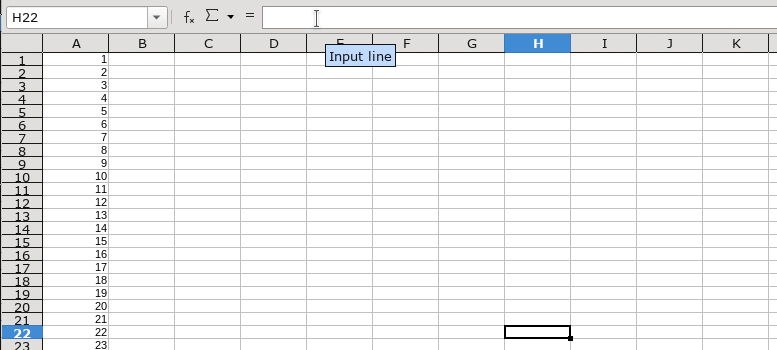# Split long column in excel

i have a column that contain 5000 cell is there any speed way to split this column to 10 other column and not doing this manual

split long column in excel

I don’t know how to do in Excel, but there is a solution for Calc - see below.

Hello,

Assumptions (for the description)

• Current data are in range `A1:A5000` (5000 rows)
• You want to get the values into 10 columns (resulting in `5000 / 10 = 500` rows)
• Target range starts in `B1` (hence in total is `B1:K500`

• Move to cell `B1`
• Add the following formula into `B1`: `=OFFSET(\$A\$1;(COLUMN()-1)+(ROW()-1)*10-1;0)`
• Drag the formula from `B1 -> K1`
• Type `B1:K500` into the Name Box (leftmost in the formula bar) and press `ENTER` (the range `B1:K500` gets selected)
• Type `CTRL+D`
• Optional Select columns `B` through `D` and `Data -> Calculate -> Formula to Value`• the starting cell of the target range is not `B1` and/or
• the first cell of the current data is not `A1` and/or
• the number of columns the data should be distributed to is not `10`

(i.e. in any case the assumptions are not fulfilled)

Hope that helps.

thank you very much this is very helpful

This way to divide it horizontally, is there a way to solve the issue vertically

What do you mean by that (`1` through `10` in `B1:B10`; `11` through `20` in `C1:C10` and so on)? If - yes, that’s of course possible, but keep in mind that due to a limit of 1024 columns (16k limit in version 7 still an experimental feature), you’ll reach the limit pretty soon (Formula: `=OFFSET(\$A\$1;(COLUMN()-2)*10+(ROW())-1;0)` and range: `B1:SG10` (add the formula to `B1`,copy [`CTRL+C`] the formula, select the range via Name Box and paste [`CTRL+V`] the formula into the range).

yes that what i means
thanks again